﻿ Java exercises: Create the first twenty Hamming numbers - w3resource# Java Exercises: Create the first twenty Hamming numbers

## Java Numbers: Exercise-27 with Solution

Write a Java program to create the first twenty Hamming numbers.

Pictorial Presentation:In computer science, regular numbers are often called Hamming numbers, Hamming Numbers are numbers whose only prime factors are 2, 3 and 5.
The first few hamming numbers are :
1, 2, 3, 4, 5, 6, 8, 9, 10, 12, 15, 16, 18, 20, 24, 25, 27, 30, 32

Sample Solution:

Java Code:

``````// https://rosettacode.org/
import java.math.BigInteger;
public class Exercise28 {

public static void main(String args[])
{
Stream hamming = makeHamming();
System.out.print("First Twenty Hamming numbers: ");
for (int i=0; i<20; i++) {
System.out.print(hamming.value());
System.out.print(" ");
}
System.out.println();
}

public interface Stream
{
BigInteger value();
}

public static class MultStream implements Stream
{
MultStream(int mult)
{ m_mult = BigInteger.valueOf(mult); }
MultStream setBase(Stream s)
{ m_base = s; return this; }
public BigInteger value()
{ return m_mult.multiply(m_base.value()); }

private final BigInteger m_mult;
private Stream m_base;
}

private final static class RegularStream implements Stream
{
RegularStream(Stream[] streams, BigInteger val)
{
m_streams = streams;
m_val = val;
}
public BigInteger value()
{ return m_val; }

{
// memoized value for the next stream instance.

int minidx = 0 ;
BigInteger next = nextStreamValue(0);
for (int i=1; i<m_streams.length; i++) {
BigInteger v = nextStreamValue(i);
if (v.compareTo(next) < 0) {
next = v;
minidx = i;
}
}
RegularStream ret = new RegularStream(m_streams, next);
// memoize the value!
return ret;
}
private BigInteger nextStreamValue(int streamidx)
{
// skip past duplicates in the streams we're merging.
BigInteger ret = m_streams[streamidx].value();
while (ret.equals(m_val)) {
ret = m_streams[streamidx].value();
}
return ret;
}
private final Stream[] m_streams;
private final BigInteger m_val;
}

private final static Stream makeHamming()
{
MultStream nums[] = new MultStream[] {
new MultStream(2),
new MultStream(3),
new MultStream(5)
};
Stream ret = new RegularStream(nums, BigInteger.ONE);
for (int i=0; i<nums.length; i++) {
nums[i].setBase(ret);
}
return ret;
}
}
```
```

Sample Output:

```First Twenty Hamming numbers: 1 2 3 4 5 6 8 9 10 12 15 16 18 20 24 25 27 30 32 36
```

Flowchart: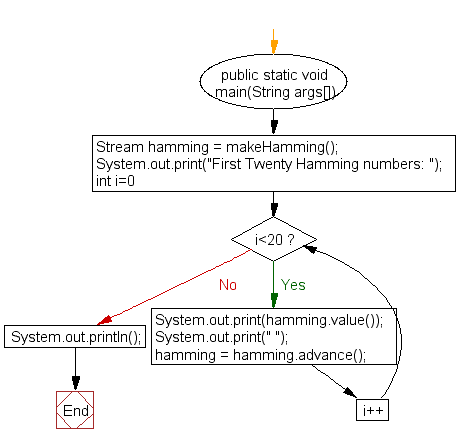interface Stream :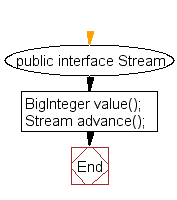MultStream implements Stream :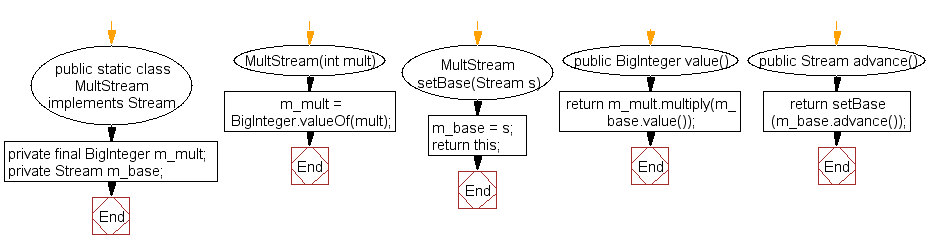RegularStream implements Stream :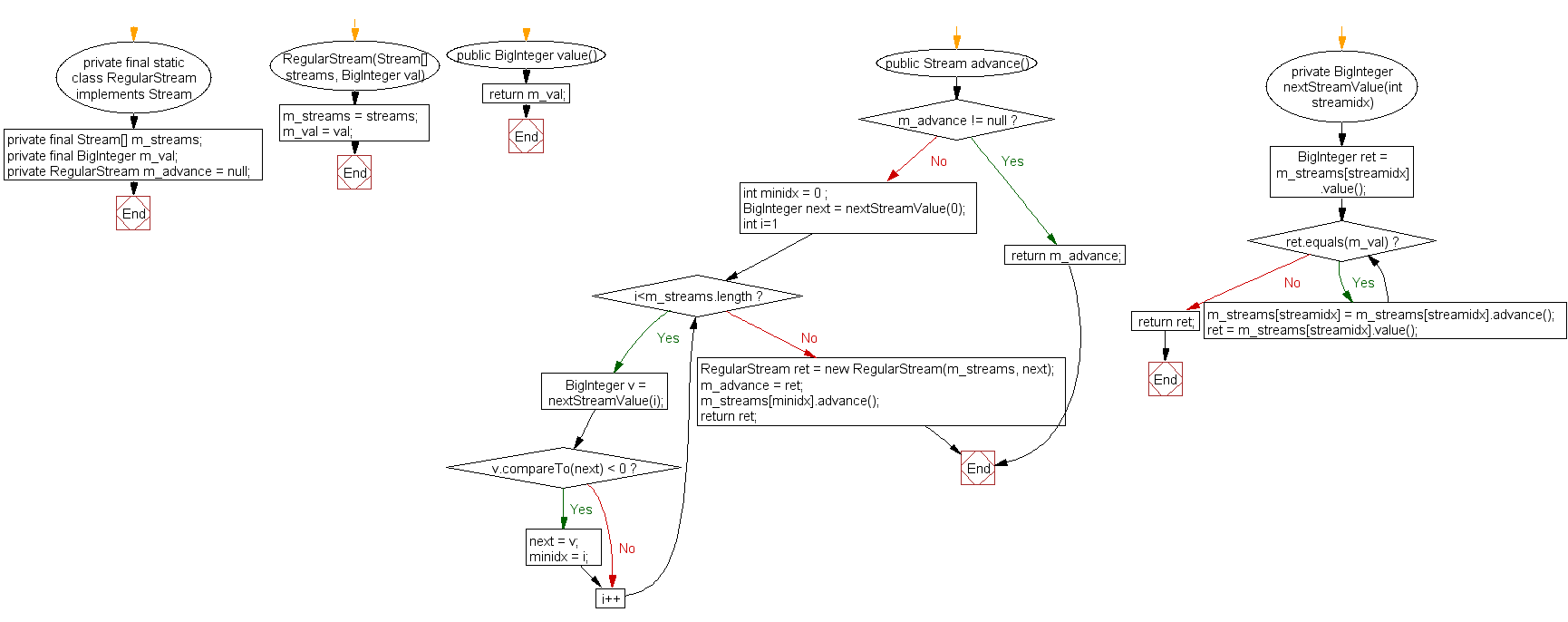Stream makeHamming :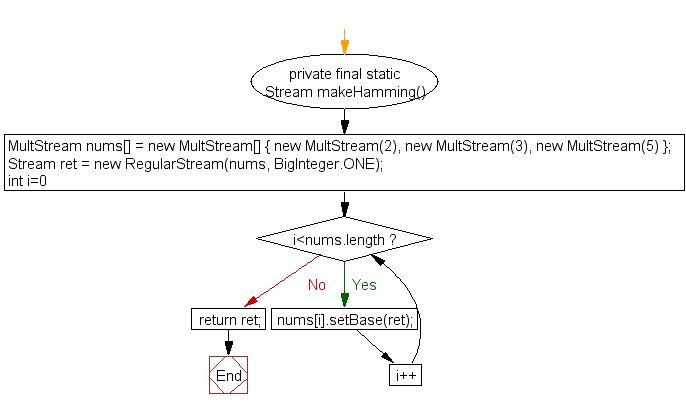Java Code Editor:

What is the difficulty level of this exercise?

﻿

## Java: Tips of the Day

Array vs ArrayLists:

The main difference between these two is that an Array is of fixed size so once you have created an Array you cannot change it but the ArrayList is not of fixed size. You can create instances of ArrayLists without specifying its size. So if you create such instances of an ArrayList without specifying its size Java will create an instance of an ArrayList of default size.

Once an ArrayList is full it re-sizes itself. In fact, an ArrayList is internally supported by an array. So when an ArrayList is resized it will slow down its performance a bit as the contents of the old Array must be copied to a new Array.

At the same time, it's compulsory to specify the size of an Array directly or indirectly while creating it. And also Arrays can store both primitives and objects while ArrayLists only can store objects.

Ref: https://bit.ly/3o8L2KH